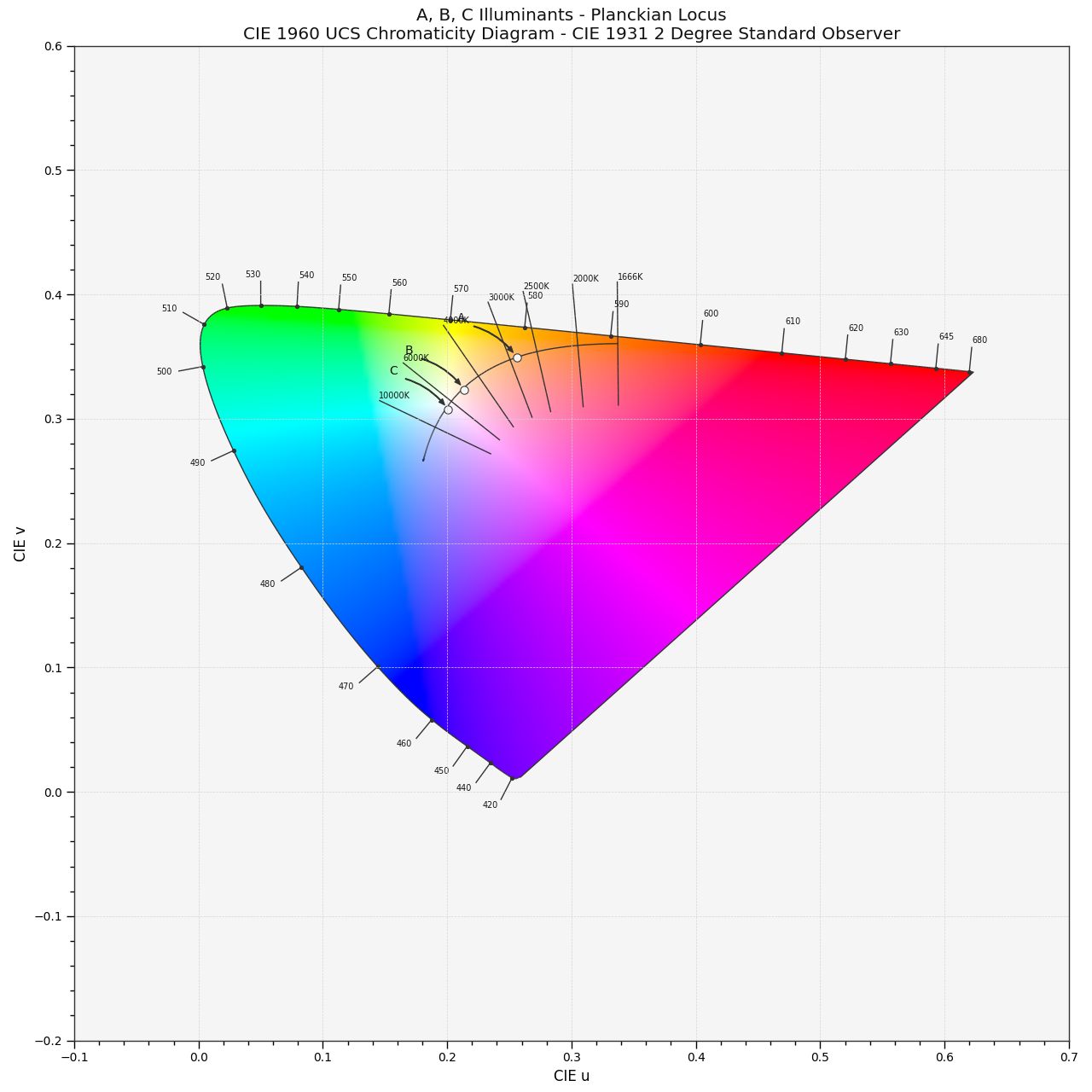# colour.plotting.plot_planckian_locus_in_chromaticity_diagram_CIE1960UCS#

colour.plotting.plot_planckian_locus_in_chromaticity_diagram_CIE1960UCS(illuminants: , chromaticity_diagram_callable_CIE1960UCS: Callable = plot_chromaticity_diagram_CIE1960UCS, annotate_kwargs: dict | List[dict] | None = None, plot_kwargs: dict | List[dict] | None = None, **kwargs: Any) [source]#

Plot the Planckian Locus and given illuminants in CIE 1960 UCS Chromaticity Diagram.

Parameters:
Returns:

Current figure and axes.

Return type:

`tuple`

Examples

```>>> plot_planckian_locus_in_chromaticity_diagram_CIE1960UCS(
...     ["A", "C", "E"]
... )
(<Figure size ... with 1 Axes>, <...Axes...>)
```